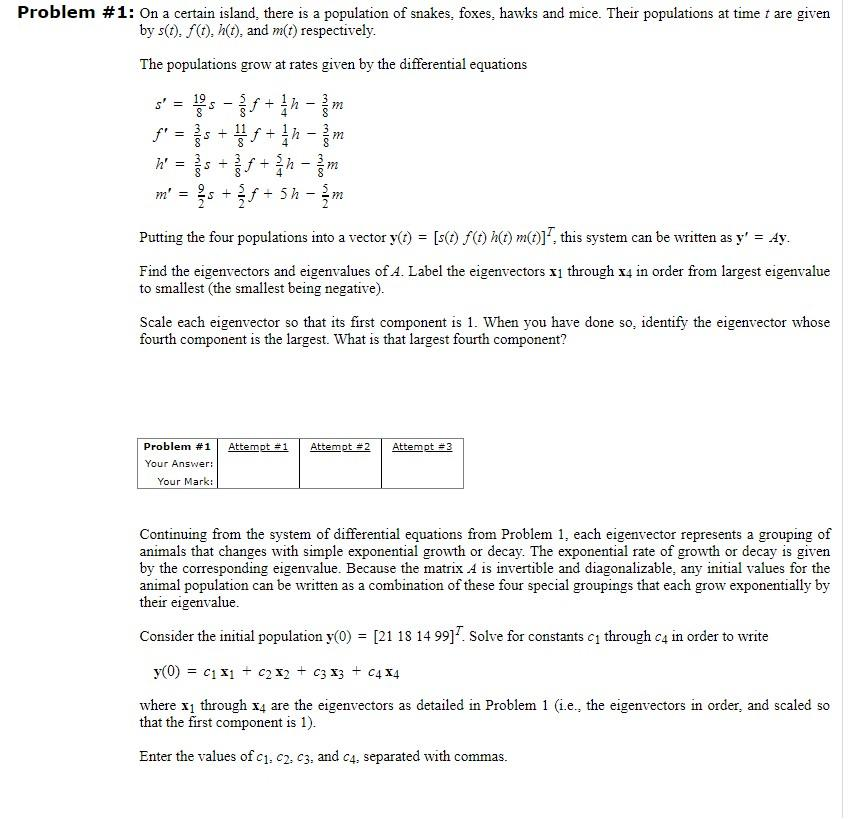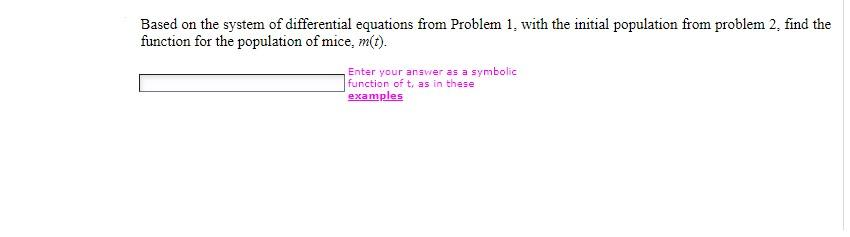# Question MatLab is recommend Problem #1: On a certain island, there is a population of snakes, foxes, hawks and mice. Their populations at time t are given by s(t). fo.h(t), and m(t) respectively. The populations grow at rates given by the differential equations s' = S- + 8 = s + + h' = S - + 11 = - - m' = s + t + 5h - f - žm Putting the four populations into a vector y(t) = [s(t) f(t) h(t) m(t)]*, this system can be written as y' = Ay. Find the eigenvectors and eigenvalues of A. Label the eigenvectors x1 through x4 in order from largest eigenvalue to smallest (the smallest being negative). Scale each eigenvector so that its first component is 1. When you have done so, identify the eigenvector whose fourth component is the largest. What is that largest fourth component? Attempt #1 Attempt = 2 Attempt #3 Problem #1 Your Answer: Your Mark: Continuing from the system of differential equations from Problem 1, each eigenvector represents a grouping of animals that changes with simple exponential growth or decay. The exponential rate of growth or decay is given by the corresponding eigenvalue. Because the matrix A is invertible and diagonalizable, any initial values for the animal population can be written as a combination of these four special groupings that each grow exponentially by their eigenvalue. Consider the initial population y(0) = [21 18 14 9917 Solve for constants C1 through c4 in order to write = y(0) = C1 X1 + 2x2 + c3 13 + C4X4 where 11 through x4 are the eigenvectors as detailed in Problem 1 (i.e., the eigenvectors in order, and scaled so that the first component is 1). Enter the values of C1,C2,C3, and C4, separated with commas. Based on the system of differential equations from Problem 1. with the initial population from problem 2. find the function for the population of mice, m(t). Enter your answer as a symbolic function of t, as in these examples